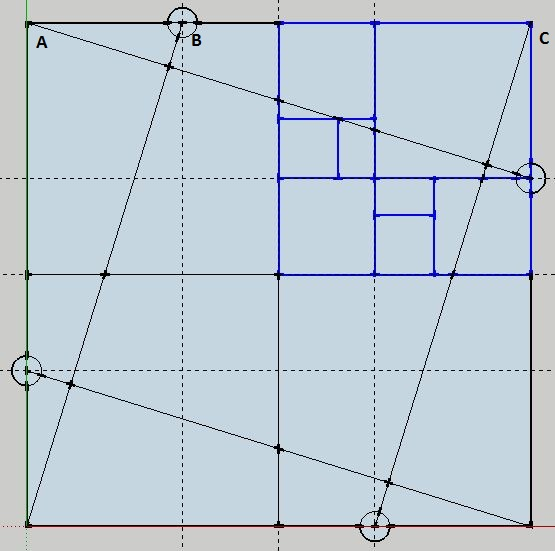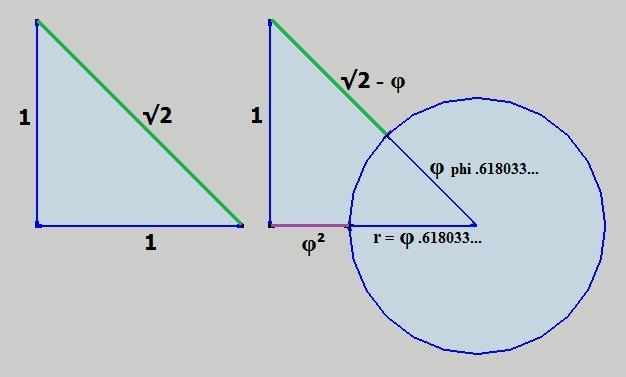Home | Study Links | Rhymes |

## 48 Golden Ratio Spirals

How to construct a perfectly harmonized Cube and Sphere:First, draw 8 golden rectangles beginning with this self-explanatory geometric configuration:
A simple 2 x 2 square, where AB= φ .618... and BC= φ .618...² + 1Here 8 golden rectangles and their converging points form a circle, and it represents one
face of a cube. Therefore, 48 golden rectangles (logarithmic spirals) signify a sphere within
the cube, and it shows a unique and fixed relationship between this Cube and Sphere.
To simplify the idea:Volume Calculations:

Cube 2³ = 8
Sphere 4/3πr³ (r = φ .618...) = .98883923...Volume 1 (V1)

8 / .98883923...(V1) = 8.09029389... x φ .618... = 5.00007660...
What if:
8 / .98885438...(V2) = 8.09016994... x φ .618... = 5 (has a slightly altered fraction 4/3)

1.6 / ϕ = 0.98885438...(V2) / π / r³ (r = φ .618...)= 1.33335376...(the new "4/3")

This Volume formula represents an unrestricted Sphere:

V = (√1.25 + 1.5) x 1.6 x r³
V = [ (√1.25 + 1.5) x 1.6 ] or [ π x 1.33335376... ] or [ (1.6 / ϕ) + 3.2 ] x r³

In this example Volumes for a Cube and Sphere of equal diameters:

Cube (side = .618... x 2) ³ = 1.8885438...
Sphere (1.6 / ϕ) = 0.98885438...

1.8885438... / 0.98885438... = 1.90983005... [ 5 / (ϕ + 1) ]
And 0.98885438... - (1.8885438... / 10) = 0.8
8 / 1.90983005... = 4.18885438... [ (1.6 / ϕ) + 3.2 ]

A Sphere in a Cylinder:

Imagine a cylinder volume πr²h ( r = φ .618... and h = 2 x r ) which contains
a sphere with the common formula 4/3πr³ (r = φ .618...) V = .98883923...
Cylinder V 1.48325884... / Sphere V .98883923... = 1.5 Here the fraction 4/3
reduced the sphere size, so as to accommodate and keep the cylinder volume!

Explanation for the two different Sphere volumes:

Sphere one 0.98883923...(V1) is restricted and the second 0.98885438...(V2) is not.
Sphere one is of slightly lesser volume because it is surrounded and "compressed"
within a "Cube", whereas the second sphere in a free state is not. Imagine a sphere
within a cube of equal diameters, where one or the other has to give up space.
So, the formula V = (√1.25 + 1.5) x 1.6 x r³ consisting of true numbers pi and phi,
represents spheres that exist in a perfectly harmonious, free and balanced state.
(Imagine a filled balloon and that same shape without the balloon material)